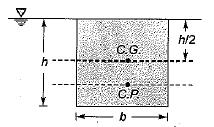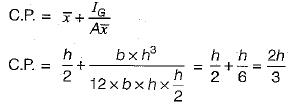Courses

# Test: Hydrostatic Forces on Surfaces

## 10 Questions MCQ Test Topicwise Question Bank for Mechanical Engineering | Test: Hydrostatic Forces on Surfaces

Description
This mock test of Test: Hydrostatic Forces on Surfaces for Mechanical Engineering helps you for every Mechanical Engineering entrance exam. This contains 10 Multiple Choice Questions for Mechanical Engineering Test: Hydrostatic Forces on Surfaces (mcq) to study with solutions a complete question bank. The solved questions answers in this Test: Hydrostatic Forces on Surfaces quiz give you a good mix of easy questions and tough questions. Mechanical Engineering students definitely take this Test: Hydrostatic Forces on Surfaces exercise for a better result in the exam. You can find other Test: Hydrostatic Forces on Surfaces extra questions, long questions & short questions for Mechanical Engineering on EduRev as well by searching above.
QUESTION: 1

Solution:
QUESTION: 2

### A rectangular water tank, full to the brim, has its length, breadth and height in the ratio 2 : 1 :2 .The ratio of hydrostatic forces on the bottom to that on any larger vertical surface of the tank is

Solution: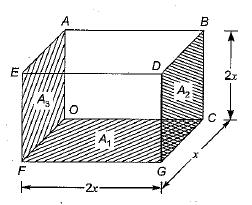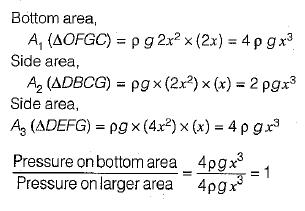QUESTION: 3

### A plane area in the form of a right angle triangle of height h base b is immersed vertically in water with its vertex at the water surface. The coordinate of the location of center of pressure IS

Solution: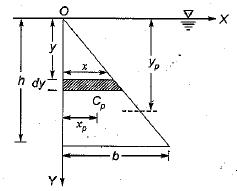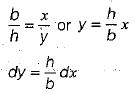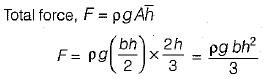Taking moments of force on the element about O Y and integrating: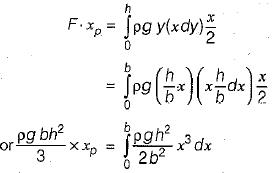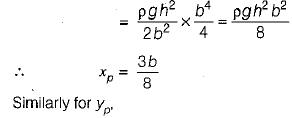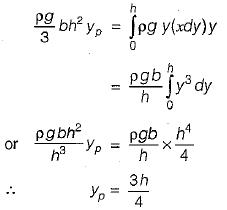so, the coordinate of center of pressure is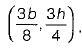QUESTION: 4

A circular disc of diameter D is immersed vertically in a liquid of density p. The top most point of the disc just touches the liquid surface . What is depth of center of pressure ?

Solution: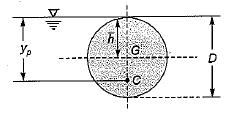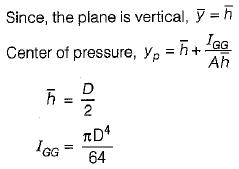QUESTION: 5

A circular annular area of inner and outer diameter, D and 2D respectively is immersed vertically in water with the center of the area at 3D below the water surface . The location of the center of pressure is

Solution: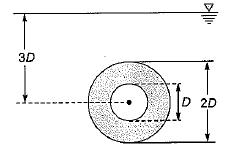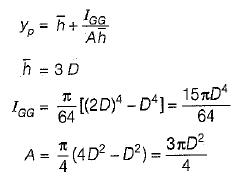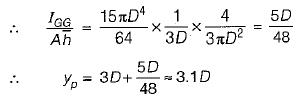QUESTION: 6

A rectangular gate is to open just as the water level reaches a height of 4 m from the bottom of the gate as shown in figure .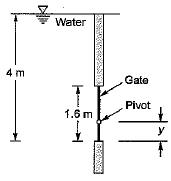What s the location  y of the pivot ? ( Take width of gate as 1 m)

Solution:

The gate will open just when the center of pressure coincides with the location of the pivot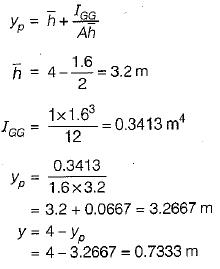QUESTION: 7

A hemispherical bulge of diameter 1.5 m is provided in the bottom of a tank. If the depth of water above the horizontal floor of the tank is 5.0 m, what is the magnitude and direction of the resultant force on the hemisphere?

Solution: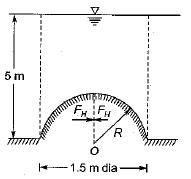Net horizontal force = 0
Fv =  weight of fluid above the hemisphere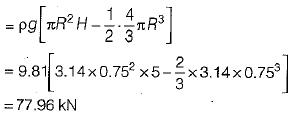QUESTION: 8

The total pressure on a horizontally immersed surface is

Where,
w = specific weight of the liquid
A = area of the immersed surface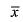=depth of the center of gravity of the immersed surface from the liquid surface

Solution: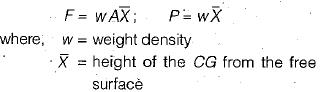QUESTION: 9

Point of application of a horizontal force on curved surface submerged in liquid is

Solution: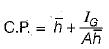where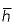is distance of centre of gravity from free surface and IG area moment of inertia

QUESTION: 10

The depth of centre of pressure for a rectangular lamina immersed vertically in water up to height h is given by

Solution: